# Angular Velocity and Acceleration

Definition: Angular Velocity

Angular velocity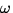of a rigid body is the rate of change of its angular position. Thus, if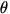=at t = t1 , and=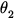at t = t2 , then the average angular velocity of the body over the time intervalt = t2 - t1 is: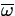=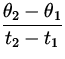=. (4)

As for linear motion, the instantaneous velocity is obtained by making the time interval very small: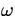=. (5)

The units of angular velocity are most conveniently given in rads/sec, but can also be expressed in revolutions/sec or degrees/sec using the conversions given above.

Definition: Angular Acceleration

Angular Acceleration is the rate of change of angular velocity with time. The average angular acceleration of a rigid body over a time intervalt = t2 - t1 is:= = . (6)

The instantaneous angular acceleration is obtained by taking a very small time interval:=. (7)

The units of angular acceleration are normally radians/sec 2.Next: Formulae for Constant Angular Up: Circular Motion and the Previous: Introduction